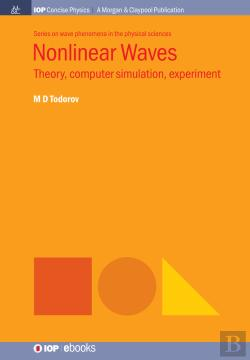Nonlinear Waves (eBook)

Theory, Computer Simulation, Experiment

de M D Todorov

idioma: Inglês
Edição ou reimpressão: setembro de 2018
55,49€

The Boussinesq equation is the first model of surface waves in shallow water that considers the nonlinearity and the dispersion and their interaction as a reason for wave stability known as the Boussinesq paradigm. This balance bears solitary waves that behave like quasi-particles. At present, there are some Boussinesq-like equations. The prevalent part of the known analytical and numerical solutions, however, relates to the 1d case while for multidimensional cases, almost nothing is known so far. An exclusion is the solutions of the Kadomtsev-Petviashvili equation. The difficulties originate from the lack of known analytic initial conditions and the nonintegrability in the multidimensional case. Another problem is which kind of nonlinearity will keep the temporal stability of localized solutions.

The system of coupled nonlinear Schroedinger equations known as well as the vector Schroedinger equation is a soliton supporting dynamical system. It is considered as a model of light propagation in Kerr isotropic media. Along with that, the phenomenology of the equation opens a prospect of investigating the quasi-particle behavior of the interacting solitons. The initial polarization of the vector Schroedinger equation and its evolution evolves from the vector nature of the model. The existence of exact (analytical) solutions usually is rendered to simpler models, while for the vector Schroedinger equation such solutions are not known. This determines the role of the numerical schemes and approaches. The vector Schroedinger equation is a spring-board for combining the reduced integrability and conservation laws in a discrete level.

The experimental observation and measurement of ultrashort pulses in waveguides is a hard job and this is the reason and stimulus to create mathematical models for computer simulations, as well as reliable algorithms for treating the governing equations. Along with the nonintegrability, one more problem appears here - the multidimensionality and necessity to split and linearize the operators in the appropriate way.

Nonlinear Waves
Theory, Computer Simulation, Experiment
de M D Todorov
ISBN: 9781643270463 Ano de edição ou reimpressão: 09-2018 Editor: MORGAN & CLAYPOOL PUBLISHERS Idioma: Inglês Tipo de Produto: eBook Formato: ePUB i Classificação Temática:

Sugestões

138,81€
INSTITUTE OF PHYSICS PUBLISHING
59,63€
CRC PRESS
X
O QUE É O CHECKOUT EXPRESSO?

O ‘Checkout Expresso’ utiliza os seus dados habituais (morada e/ou forma de envio, meio de pagamento e dados de faturação) para que a sua compra seja muito mais rápida. Assim, não tem de os indicar de cada vez que fizer uma compra. Em qualquer altura, pode atualizar estes dados na sua ‘Área de Cliente’.

Para que lhe sobre mais tempo para as suas leituras.